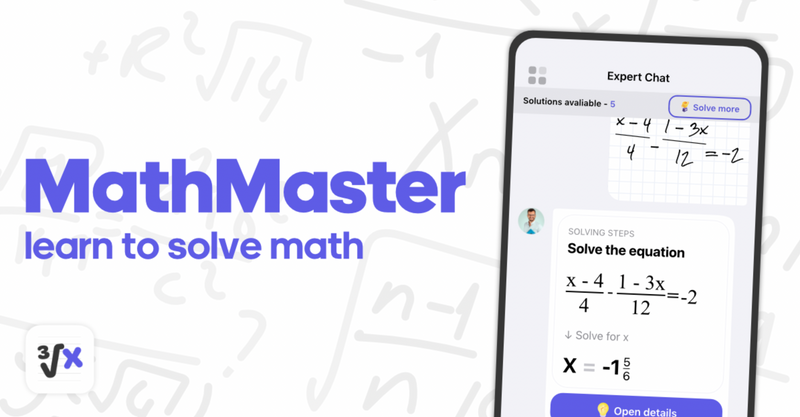### Math Has Never Been Easier with MathMaster

The app helps solve math and teaches students how to do their math homework fast and correctly, all with just a phone camera.

United States – February 3, 2023MathMaster is a math problem solver tool that provides outstanding math homework assistance. The app is a step-by-step math solver trusted by thousands of students worldwide.

It is found that 93 percent of adults in the US have experienced some anxiety over math, while around half of high schoolers have “very high math anxiety.” The MathMaster app aims to make math “accessible and fun” for everyone.

MathMaster helps to do the math by just using a camera – snap a picture of a math problem or load an image, and get the right answer on the screen. For a better understanding of how to solve a problem – just check a step-by-step solution.

MathMaster covers all levels of math from Basic Math/Pre-algebra (arithmetic, integers, fractions, decimal numbers, powers, roots, factors), Algebra (linear equations/inequalities, quadratic equations, systems of equations, logarithms, functions, matrices, graphing, polynomials), Trigonometry/Precalculus (identities, sequences and series, logarithmic functions), Calculus (limits, derivatives, integrals), and Statistics (combinations, factorials).

MathMaster recognizes both handwritten problems as well as printed ones. Just snap a problem or upload one from the photo gallery. The app also provides both straight answers and the corresponding step-by-step solutions.

“My daughter has always struggled with algebra and geometry. We decided to give MathMaster a try to see if we could improve her grades and confidence before high school. Amazing experience! Excellent math problem solving step-by-step. 10/10!” reviewed a satisfied user.

Take the learning process to another level by understanding “how” and “why” math problems are solved or remembering forgotten terms and concepts. Solve math problems instantly across a wide variety of topics, ranging from Arithmetic to Calculus. Learn from solving steps and explanations and improve your math skills daily.

MathMaster is a math problem solver tool that provides outstanding math homework assistance and is trusted by thousands of students worldwide. The app was developed by math problem-solving wizards Nadia Moore, Catherine Graham, Aaron Marcus, Jacob Furlan, Gertrude Davidson, and Stephen Mulware. Students can find solutions to all math problems ranging from Basic Math, Algebra, Pre-algebra, Trigonometry, Statistics, Calculus, and more!

Contact Info:
Name: MathMaster
Email: Send Email
Organization: MathMaster
Website: https://math-master.org/

Release ID: 89089514

If you detect any issues, problems, or errors in this press release content, kindly contact error@releasecontact.com to notify us. We will respond and rectify the situation in the next 8 hours.

Go Top